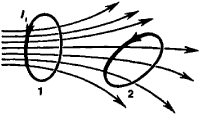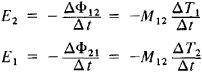# Mutual Inductance

Also found in: Dictionary, Thesaurus, Wikipedia.

## mutual inductance

[′myü·chə·wəl in′dək·təns]
(electromagnetism)
Property of two neighboring circuits, equal to the ratio of the electromotive force induced in one circuit to the rate of change of current in the other circuit.
McGraw-Hill Dictionary of Scientific & Technical Terms, 6E, Copyright © 2003 by The McGraw-Hill Companies, Inc.
The following article is from The Great Soviet Encyclopedia (1979). It might be outdated or ideologically biased.

## Mutual Inductance

a quantity that characterizes the magnetic coupling between two or more electric circuits. If there are two conducting circuits, (1) and (2), some of the lines of magnetic induction generated by the current flowing through the first circuit will penetrate the area within the second circuit (see Figure 1)—that is, they will be coupled with the second circuit. The magnetic flux Φ12 through circuit (2) generated by the current I in circuit (1) is directly proportional to the current:

Φ12 = M12I1

The proportionality coefficient M2 depends on the dimensions and form of circuits (1) and (2), on the distance between the circuits, on their relative positions, and on the magnetic permeability of the surrounding medium. This coefficient is called mutual inductance or the coefficient of mutual induction. In the SI system of units, mutual inductance is measured in henrys.Figure 1

If current I2 flows through circuit (2), the magnetic flux Φ12 through the area of circuit (1) is also proportional to the current:

Φ21 = M21I2

where M21 = M12.

The existence of a magnetic coupling between the circuits is manifested by the fact that, upon a change of current in one circuit, an induced electromotive force appears in the adjacent circuit. According to the law of electromagnetic induction,where E2 and E1 are induced electromotive forces arising in circuits (2) and (1) and ΔΦ]2 and ΔΦ21 are the change in magnetic fluxes through the respective circuits over time Δt.

The mutual energy W12 of the magnetic field is expressed with the aid of mutual inductance for the currents I1 and I2 as follows:

Wl2 = ±M12I1I2

The sign in (4) depends on the direction of the currents.

### REFERENCE

Kalashnikov, S.G. Elektrichestvo. Moscow, 1970. Chapter 10. (Obshchiikurs fiziki, vol. 2.)

G. IA. MIAKISHEV

The Great Soviet Encyclopedia, 3rd Edition (1970-1979). © 2010 The Gale Group, Inc. All rights reserved.
References in periodicals archive ?
In general, compared to the mutual inductance strengths between TXs and the RX ([M.sub.iR]), the ones between TXs ([M.sub.ij], i, j =1, 2, ..., n, and i [not equal to] j) are small enough to be omitted, namely, [M.sub.ij] = 0.
To obtain [L.sub.oe] [22-27], we focus on the mutual inductance of a pair of parallel conductors, whose expression is:
From the steady state responses, the previously estimated resistance, R, and (10), the mutual inductance value is [k.sub.0] = 0.186 N-m/Wb-A.
In the formula(1), [u.sub.a], [u.sub.b], [u.sub.c] are terminal voltage of three-phase stator motor (V); [e.sub.a], [e.sub.b],[e.sub.c] are counter electromotive force (V) in a three-phase motor winding; R = [R.sub.a] = [R.sub.b] = [R.sub.c] are three-phase motor winding resistance ([OMEGA]); [i.sub.a], [i.sub.b], [i.sub.c] are three phase current of stator motor(A); L = [L.sub.a] = [L.sub.b] = [L.sub.c] are the inductance of three-phase windings of the motor (H); M = [L.sub.ab] = [L.sub.ac] = [L.sub.bc] = [L.sub.ba] = [L.sub.ca] = [L.sub.cb] are mutual inductance between Three-phase motor stator winding(H).
Eddy current in aircraft skin also induces magnetic field, a portion of which surrounds the cable and that is the reason why mutual inductance exists.
We extend the analysis to compute the self- and mutual inductance per unit length due to its importance role in the high-speed digital circuits or simultaneous switching noise generates disparities among local found potentials in different packages  using equation (16):
The key to fault tolerance in PM machines is to have both a sufficiently high phase inductance to limit short circuit currents and a sufficiently low mutual inductance between phases to avoid the performance degradation in the remaining healthy phases.
Mutual Inductance. The mutual inductance between a primary and secondary single turn carbon fiber coil in a concentric coplanar arrangement is computed using a DC (steady-state) model .
Figure 1 shows the equivalent circuit model of the 2-coil WPT system using magnetically coupled resonator, where the coil system with self-inductance [L.sub.1] and [L.sub.2], mutual inductance M, and copper loss [r.sub.1] and [r.sub.2].
The related interactions depend on the coupling capacitance, the mutual inductance, and the tunneling resistance, which will be discussed in the subsequent section.
The [A.sub.sc] field changes the magnetic flux in the current ring, from which inductance and mutual inductance can be derived.
The coupled inductances can be represented as a mutual inductance M, two equivalent leakage inductances [L'.sub.1] and [L'.sub.2].

Site: Follow: Share:
Open / Close